# NCERT Solutions for Class 6 Maths Chapter 12 – Ratio and Proportion

NCERT Solutions for Class 6 Maths Chapter 12 – Ratio and Proportion comprises of the 3 Exercises

This Chapter contains the Exercises relating to the following topics, which are discussed in Chapter 12 – Ratio and Proportion Class 6 NCERT book  : –

• 12.1 Introduction
• 12.2 Ratio
• 12.3 Proportion
• 12.4 Unitary Method

### NCERT Solutions for Class 6 Maths Chapter 12 Exercise 12.2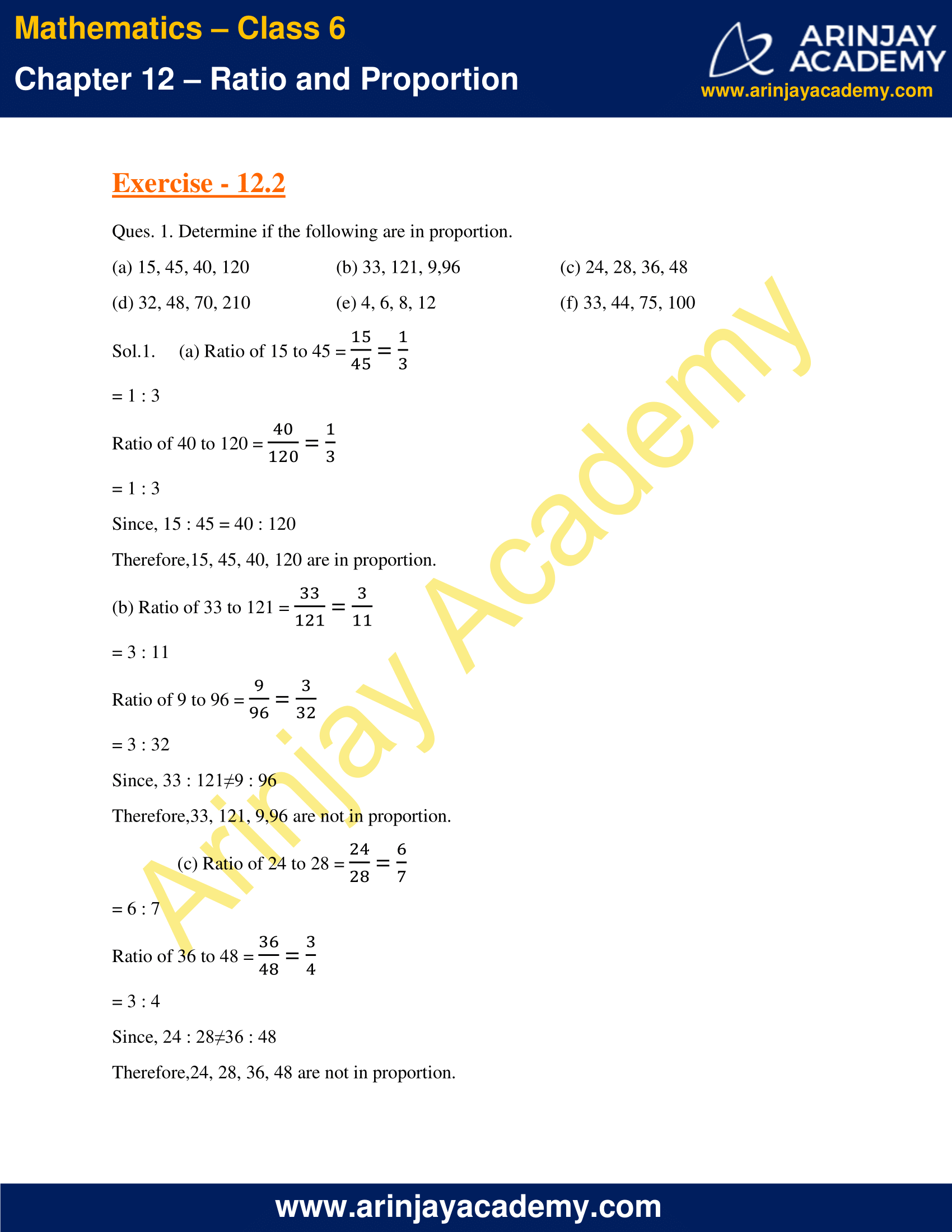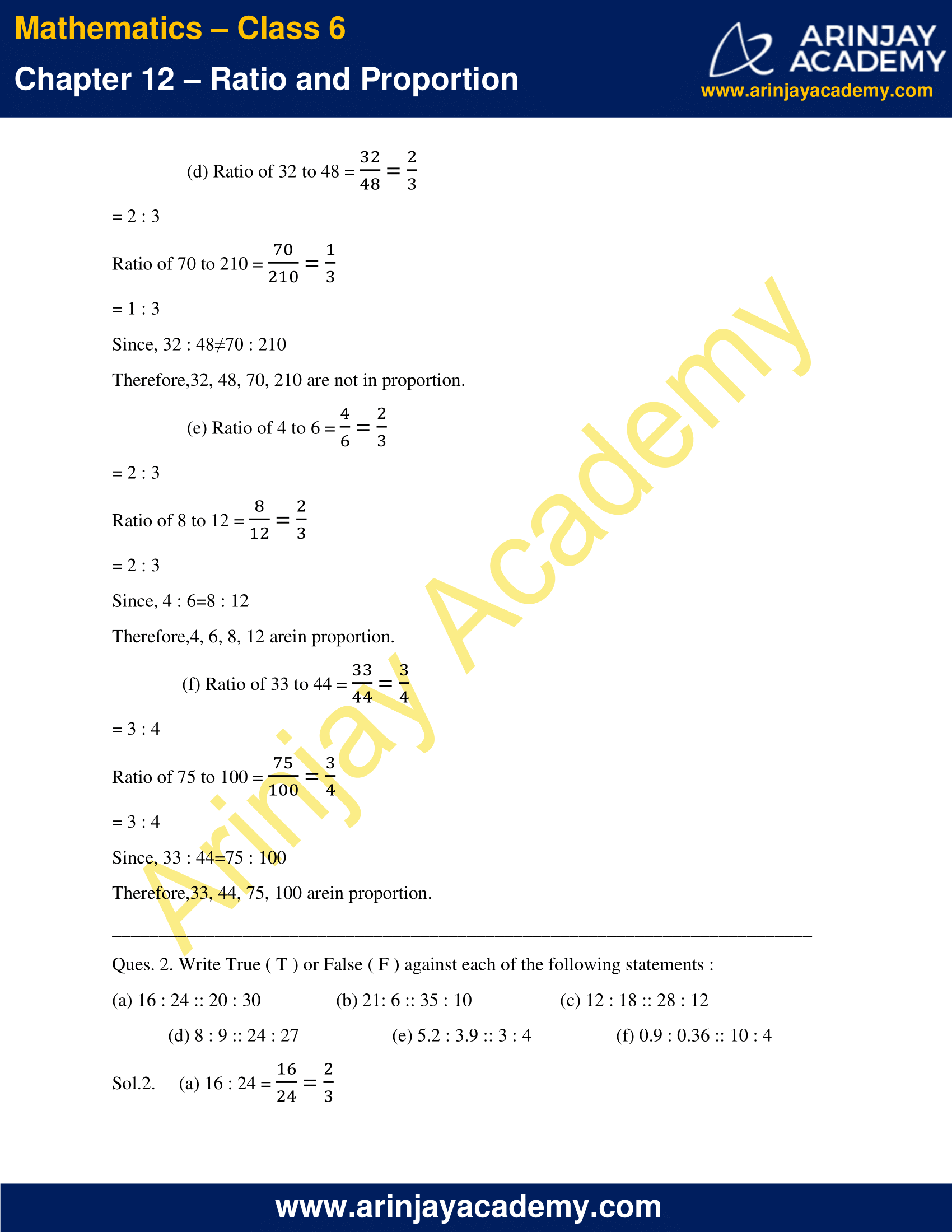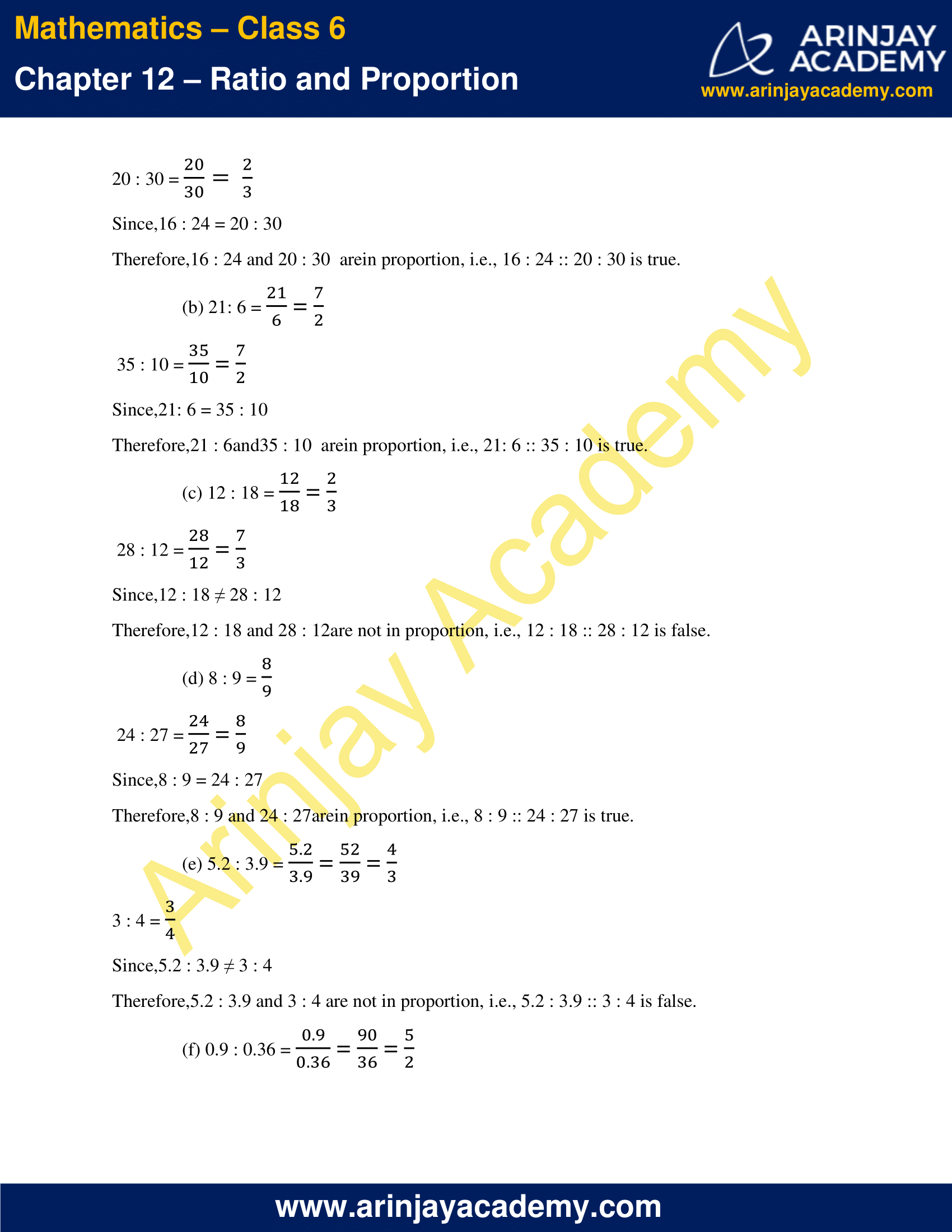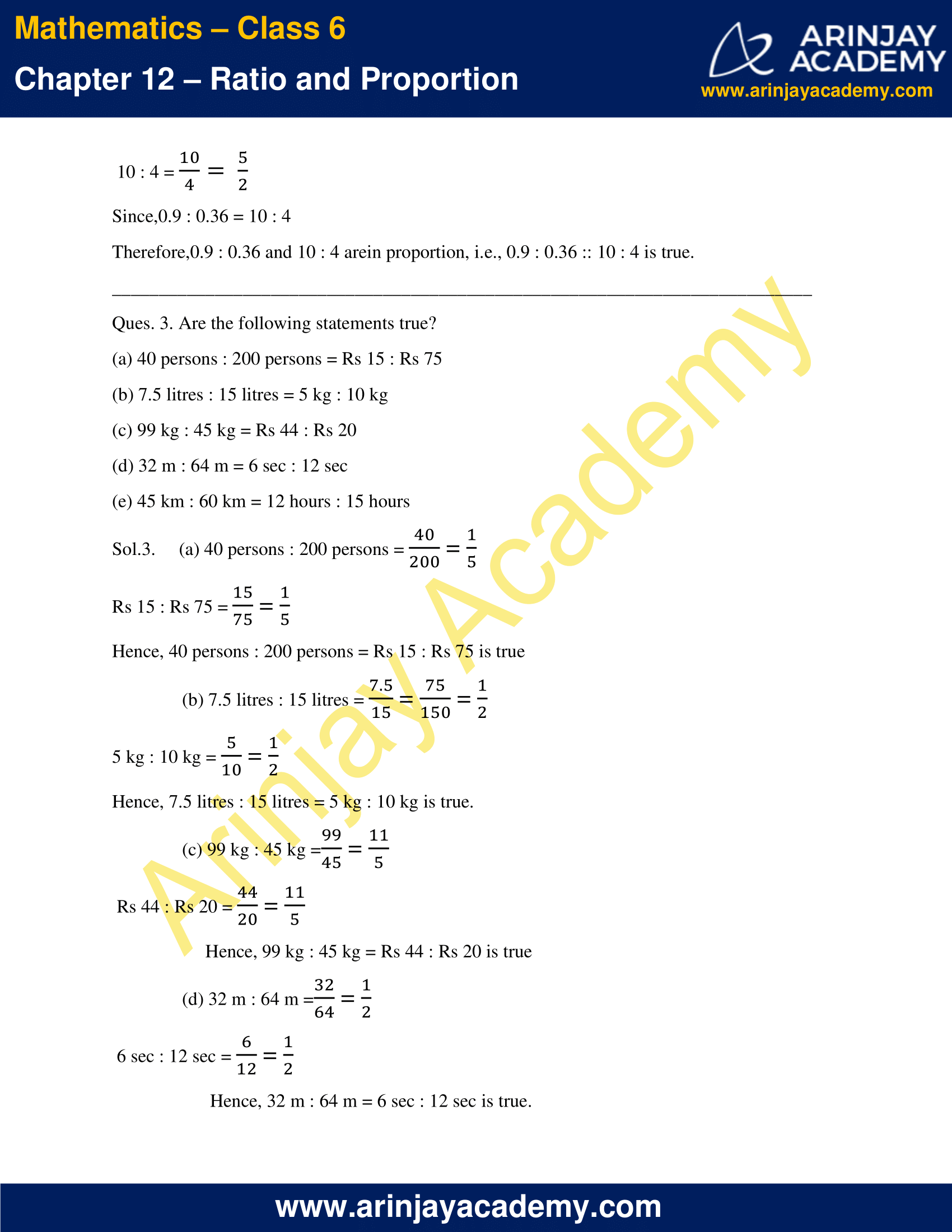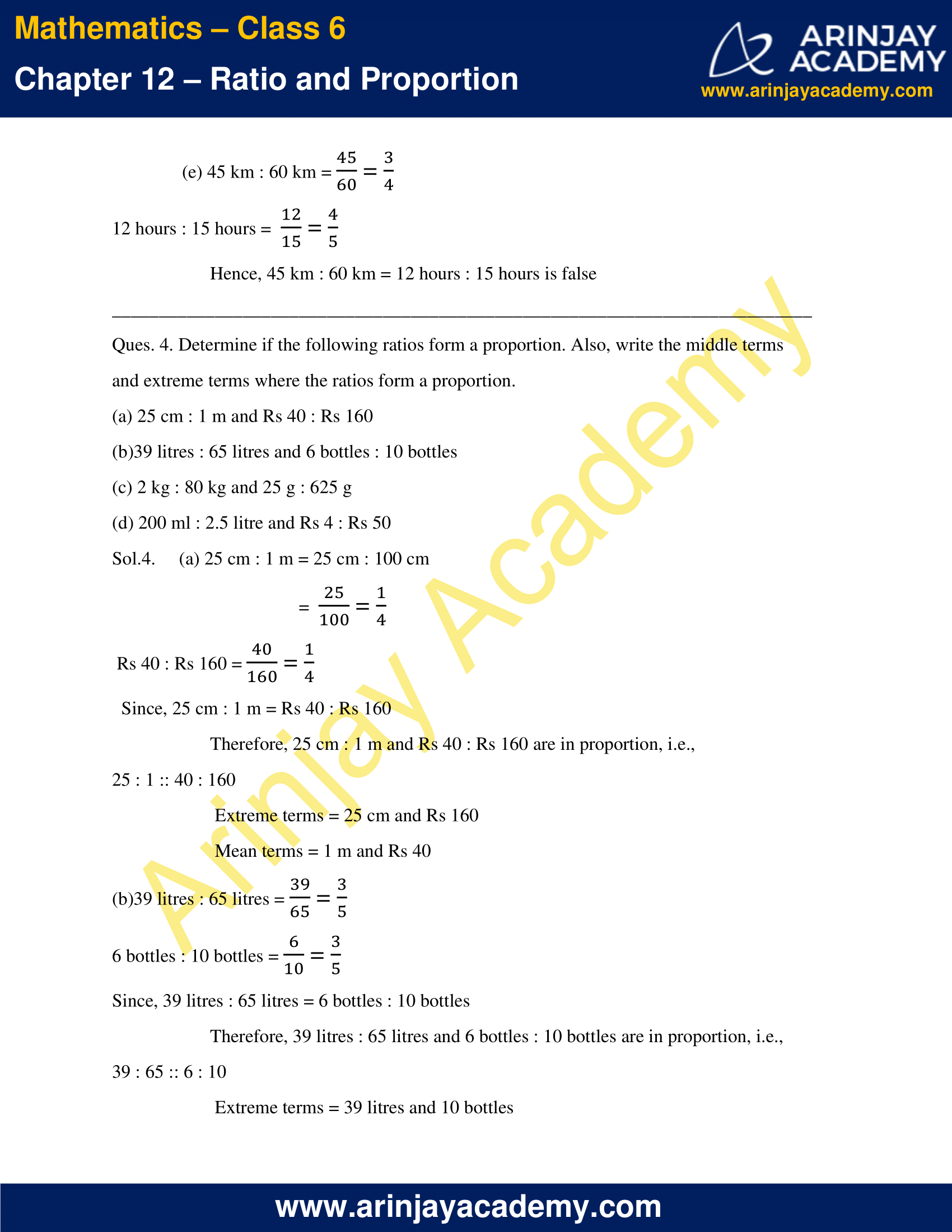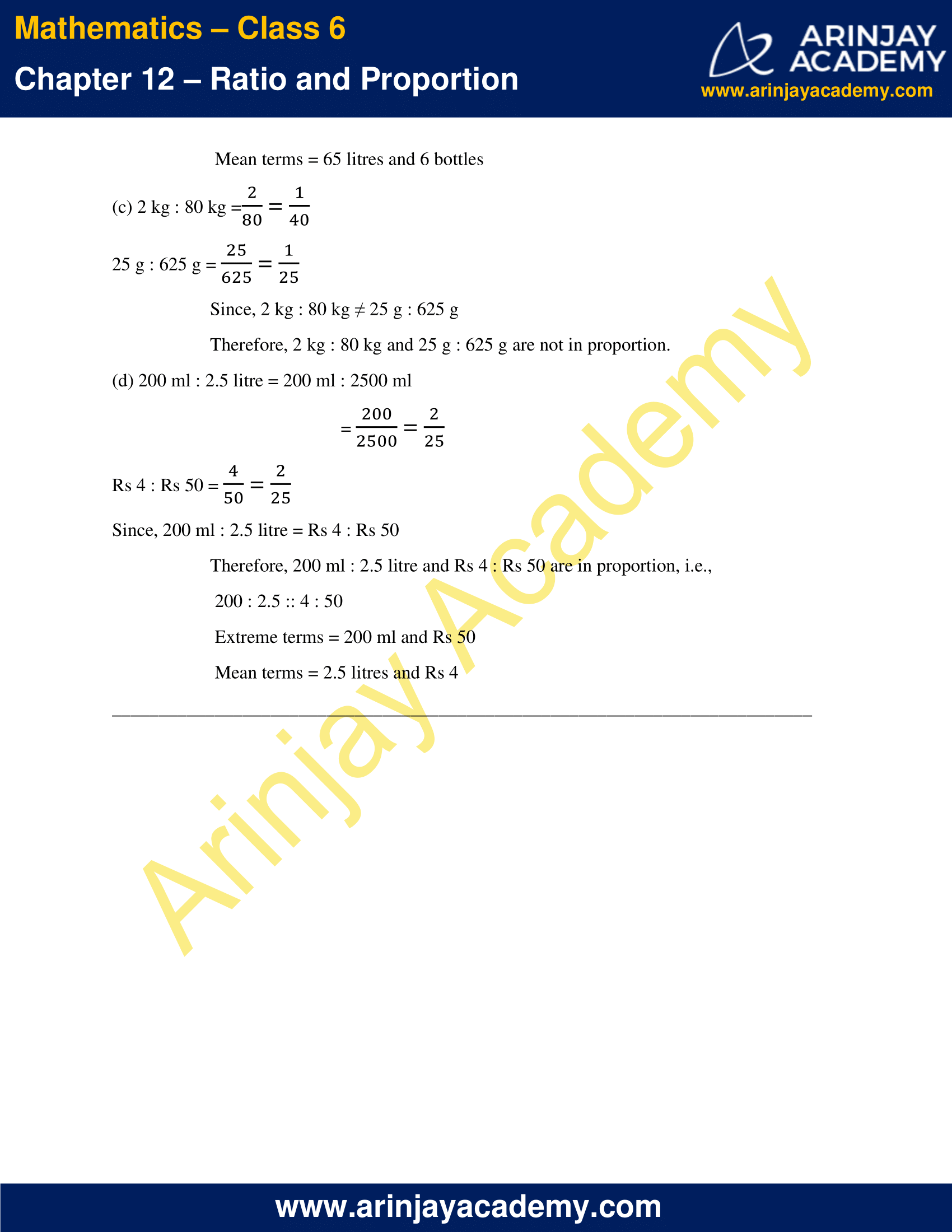### NCERT Solutions for Class 6 Maths Chapter 12 Exercise 12.3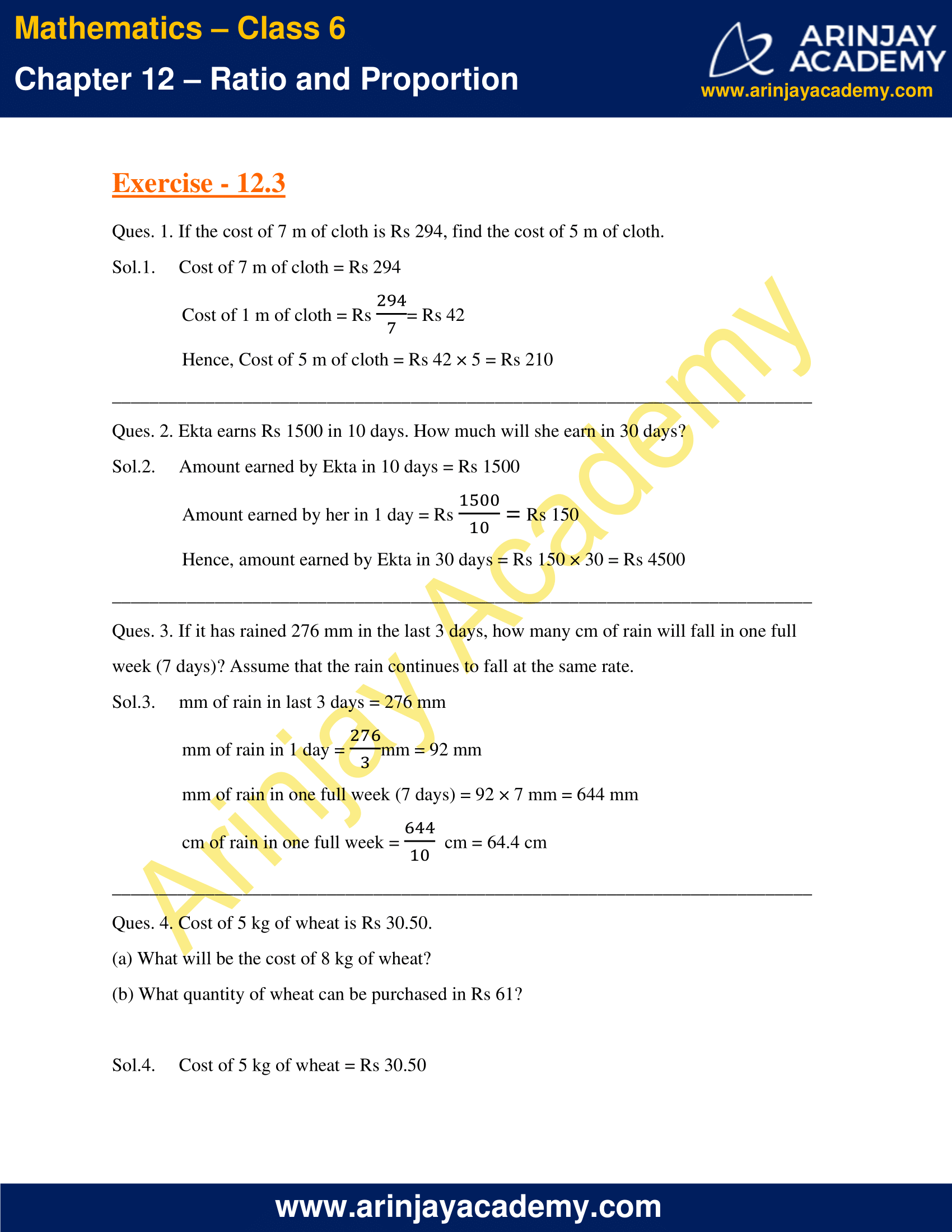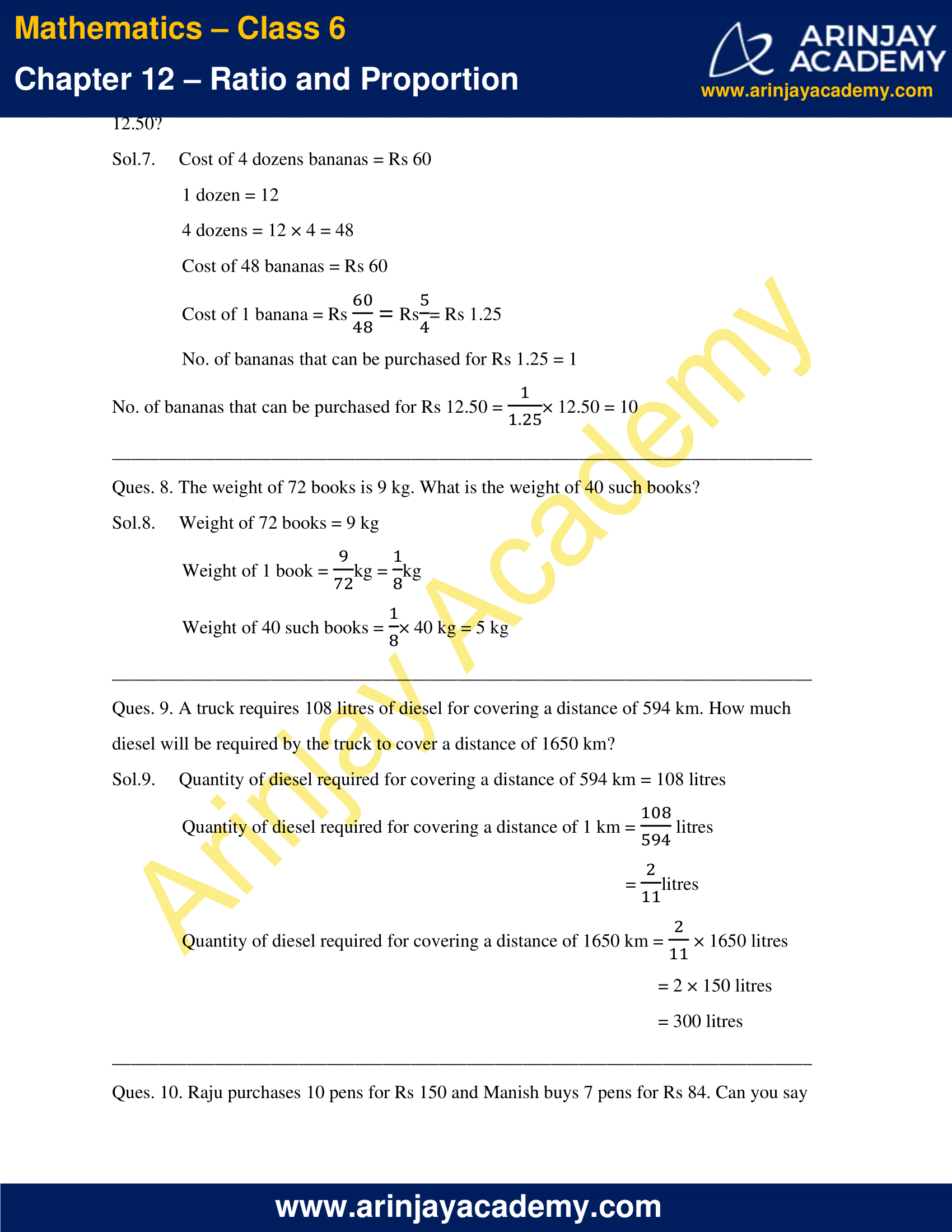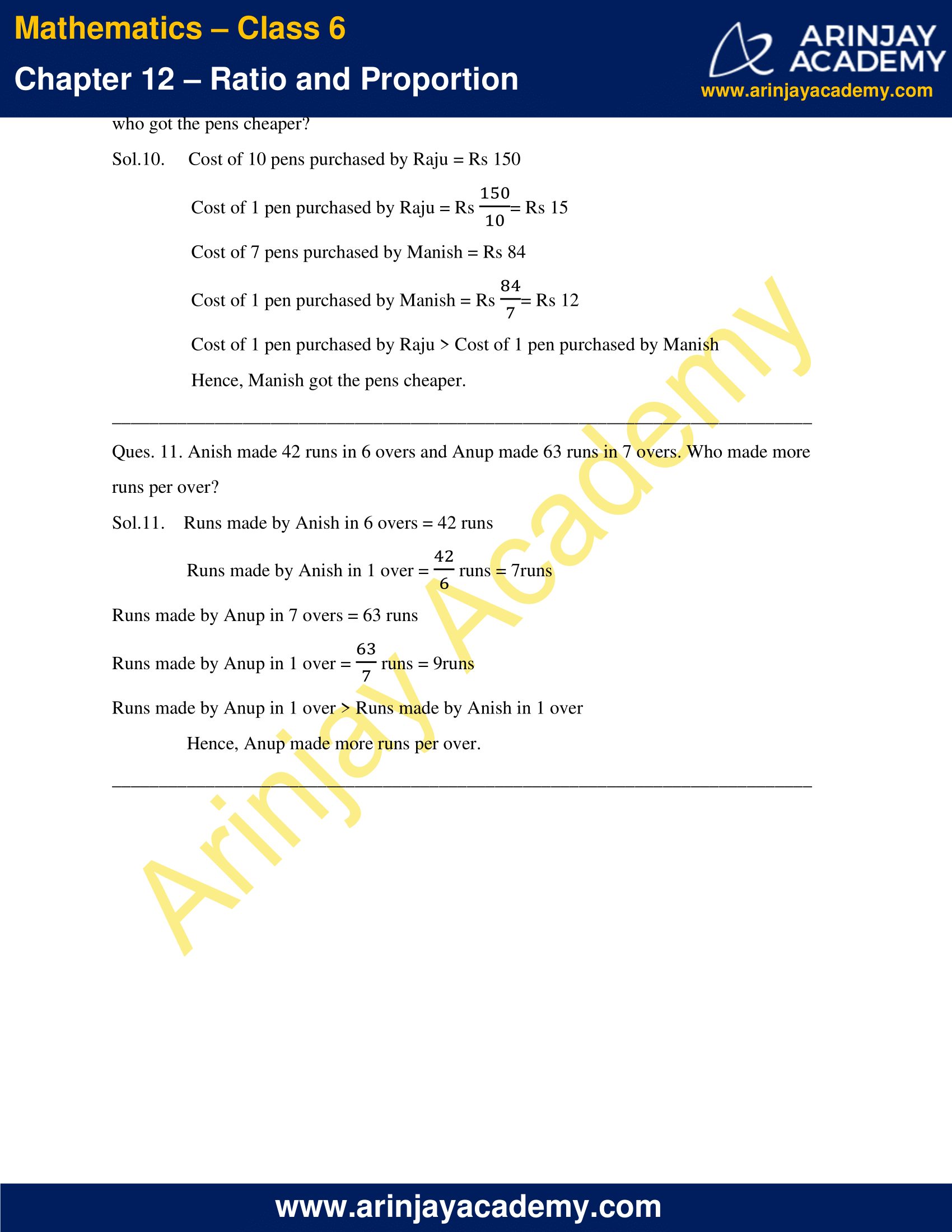### NCERT Solutions for Class 6 Maths Chapter 12 Exercise 12.1

Ques. 1. There are 20 girls and 15 boys in a class.
(a) What is the ratio of number of girls to the number of boys?
(b) What is the ratio of number of girls to the total number of students in the class?

Sol.1.  No. of girls in class = 20la
No. of boys in class = 15
Total no. of students in class = 20 + 15 = 35

(a) Ratio of number of girls to the number of boys
= No. of Girls/No. of Boys
= 20/15
= 4/3
= 4 : 3

(b) Ratio of number of girls to the total number of students in the class
= No. of Girls/Total no. of Students
= 20/35
= 4/7
= 4 : 7

Ques. 2. Out of 30 students in a class, 6 like football, 12 like cricket and remaining like tennis. Find the ratio of
(a) Number of students liking football to number of students liking tennis.
(b) Number of students liking cricket to total number of students.

Sol.2. Total no. of students in a class = 30
No. of students liking football  = 6
No. of students liking cricket   = 12
No. of students liking tennis    = 30 – (6 + 12)
= 30 – 18
= 12
(a) Ratio of no. of students liking football to no. of students liking tennis
= 6/12
= 1/2
= 1 : 2

(b) Ratio of no. of students liking cricket to total no. of students
= 12/30
= 2/5
= 2 : 5

Ques. 3. See the figure and find the ratio of
(a) Number of triangles to the number of circles inside the rectangle.
(b) Number of squares to all the figures inside the rectangle.
(c) Number of circles to all the figures inside the rectangle.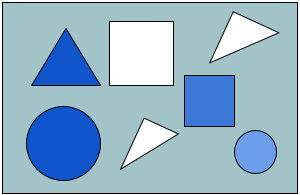Sol.3. No. of triangles inside the rectangle = 3
No. of squares inside the rectangle = 2
No. of circles inside the rectangle = 2
Total no. of figures inside the rectangle = 3 + 2 + 2 = 7

(a) Ratio of number of triangles to the number of circles inside the rectangle
= 3/2
= 3 : 2

(b) Ratio of number of squares to all the figures inside the rectangle
= 2/7
= 2 : 7

(c) Ratio of number of circles to all the figures inside the rectangle.
= 2/7
= 2 : 7

Ques. 4. Distances travelled by Hamid and Akhtar in an hour are 9 km and 12 km. Find the ratio of speed of Hamid to the speed of Akhtar.

Sol.4. Distance travelled by Hamid in an hour = 9 km
Distance travelled by Akhtar in an hour = 12 km
Hamid Speed = 9 km/hr
Akhtar Speed = 12 km/hr
Ratio of speed of Hamid to the speed of Akhtar
= Speed of Hamid/Speed of Akhtar
= 9/12
= 3/4
= 3 : 4

Ques. 5. Fill in the following blanks:
15/18 = __/6 = 10/__ = __/30
[Are these equivalent ratios?]

Sol.5. 15/18 = __/6 = 10/__ = __/30
15/18 = (15÷3)/(18÷3) = 5/6 …..(i)
5/6 = (5×2)/(6×2) = 10/12 …..(ii)
5/6 = (5×5)/(6×5) = 25/30 …..(iii)
From equations (i), (ii) and (iii),
15/18 = 5/6 = 10/12 = 25/30
Yes, all these are equivalent ratios.

Ques. 6. Find the ratio of the following:

(a) 81 to 108
(b) 98 to 63
(c) 33 km to 121 km
(d) 30 minutes to 45 minutes

Sol.6.  (a) 81 to 108 = 81/108 = (81÷9)/(108÷9) = 9/12 = (9÷3)/(12÷3) = 3/4
= 3 : 4

(b) 98 to 63 = 98/63 = (98÷7)/(63÷7) = 14/9
= 14 : 9

(c) 33 km to 121 km = 33/121 = (33÷11)/(121÷11) = 3/11
= 3 : 11

(d) 30 minutes to 45 minutes = 30/45 = (30÷15)/45÷15) = 2/3
= 2 : 3

Ques. 7. Find the ratio of the following:

(a) 30 minutes to 1.5 hours
(b) 40 cm to 1.5 m
(c) 55 paise to Re 1
(d) 500 ml to 2 litres

Sol.7. (a) 30 minutes to 1.5 hours = 30 minutes to (1.5 × 60) minutes
= 30 minutes to 90 minutes
= 30/90 = (30÷30)/(90÷30) = 1/3
= 1 : 3

(b) 40 cm to 1.5 m = 40 cm to (1.5 × 100) cm
= 40 cm to 150 cm
= 40/150 = (40÷10)/(150÷10) = 4/15
= 4 : 15

(c) 55 paise to Re 1 = 55 paise to (1 × 100) paise
= 55 paise to 100 paise
= 55/100 = (55÷5)/(100÷5) = 11/20
= 11 : 20

(d) 500 ml to 2 litres = 500 ml to (2 × 1000) ml
= 500 ml to 2000 ml
= 500/2000 = (500÷100)/(2000/100) = 5/20 = (5÷5)/(20/5) = 1/4
= 1 : 4

Ques. 8. In a year, Seema earns Rs 1,50,000 and saves Rs 50,000. Find the ratio of
(a) Money that Seema earns to the money she saves.
(b) Money that she saves to the money she spends.

Sol.8.    Money that Seema earns in a year = Rs 1,50,000
Money she saves  = Rs 50,000
Money she spends = Rs 1,50,000 – Rs 50,000
= Rs 1,00,000

(a) Ratio of money that Seema earns to the money she saves
150000/50000 = (150000÷10000)/(50000÷10000) = 15/5 = 3/1
= 3 : 1

(b) Ratio of money that she saves to the money she spends
50000/100000 = (50000÷10000)/(100000÷10000) = 5/10 = 1/2
= 1 : 2

Ques. 9. There are 102 teachers in a school of 3300 students. Find the ratio of the number of teachers to the number of students.

Sol.9.  No. of teachers in a school = 102
No. of students = 3300
Ratio of the number of teachers to the number of students
= No. of teachers/No. of students
= 102/3300 = 102÷6/3300÷6 = 17/550
= 17 : 550

Ques. 10. In a college, out of 4320 students, 2300 are girls. Find the ratio of

(a) Number of girls to the total number of students.
(b) Number of boys to the number of girls.
(c) Number of boys to the total number of students.

Sol.10. Total no. of students in a college = 4320
No. of girls = 2300
No. of boys = (4320 – 2300)
= 2020

(a) Ratio of number of girls to the total number of students
= No. of girls/No. of students
= 2300/4320
= (2300÷10)/(4320÷10)
= 230/432
= (230÷2)/(432÷2)
= 115/216
= 115 : 216

(b) Ratio of number of boys to the number of girls
= No. of boys/No. of girls
= 2020/2300
= (2020÷10)/(2300/10)
= 202/230
= (202÷2)/(230÷2)
= 101/115
= 101 : 115

(c) Ratio of number of boys to the total number of students
= No. of boys/No. of Students
= 2020/4320
= (2020÷10)/(4320÷10)
= 202/432
= (202÷2)/(432÷2)
= 101/216
= 101 : 216

Ques. 11. Out of 1800 students in a school, 750 opted basketball, 800 opted cricket and remaining opted table tennis. If a student can opt only one game, find the ratio of

(a) Number of students who opted basketball to the number of students who opted table tennis.
(b) Number of students who opted cricket to the number of students opting basketball.
(c) Number of students who opted basketball to the total number of students.

Sol.11. No. of students in school = 1800
No. of students who opted basketball   = 750
No. of students who opted cricket = 800
No. of students who opted table tennis = 1800 – (750 + 800)
= 1800 – 1550
=  250

(a) Ratio of no. of students who opted basketball to the no. of students who opted table tennis
= 750/250
= (750÷10)/(250÷10)
= 75/25
= (75÷5)/(25÷5)
= 15/5
= 3/1
= 3 : 1

(b) Ratio of no. of students who opted cricket to the no. of students opting basketball
= 800/750
= (800÷10)/(750÷10)
= 80/75
= (80÷5)/(75÷5)
= 16/15
= 16 : 15

(c) No. of students who opted basketball to the total no. of students
= 750/1800
= (750÷10)/(1800÷10)
= 75/180
= (75÷5)/(180÷5)
= 15/36
= (15÷3)/(36÷3)
= 5/12
= 5 : 12

Ques. 12. Cost of a dozen pens is Rs 180 and cost of 8 ball pens is Rs 56. Find the ratio of the cost of a pen to the cost of a ball pen.

Sol.12. 1 dozen = 12
Cost of a dozen pens = cost of 12 pens = Rs 180
Cost of one pen = Rs (180/12) = Rs 15
Cost of 8 ball pens = Rs 56
Cost of a ball pen = Rs (56/8) = Rs 7
Ratio of the cost of a pen to the cost of a ball pen
= Cost of a pen/Cost of a ball pen.
= 15/7
= 15 : 7

Ques. 13. Consider the statement: Ratio of breadth and length of a hall is 2 : 5. Complete the following table that shows some possible breadths and lengths of the hall.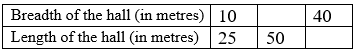Sol.13 Ratio of breadth and length of a hall is 2 : 5
If length of the hall = 50 m,
x : 50 = 2 : 5
(x/50) = (2/5)
x = (2/5) × 50 = 2 × 10 = 20 m
Hence, breadth = 20 m, if length = 50 m.
Similarly,
If breadth of the hall = 40 m,
Let length be ‘y’ m.
40 : y = 2 : 5
(40/y) = (2/5)
y = (5/2) × 40 = 5 × 20 = 100 m
Hence, length = 100 m, if breadth = 40 m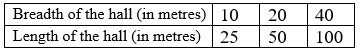Ques. 14. Divide 20 pens between Sheela and Sangeeta in the ratio of 3 : 2.

Sol.14. Let Sheela get ‘x’ number of pens. Sangeeta gets (20 – x) number of pens.
Ratio of no. of pens Sheela gets to the no. of pens Sangeeta gets = x : (20 – x)
x/(20-x) = 3/2
2x = 3 × (20 – x)
2x = 60 – 3x
2x + 3x = 60
5x = 60
x = 60/5 = 12
20 – x = 20 – 12 = 8
Hence, Sheela gets 12 pens and Sangeeta gets 8 pens.

Ques. 15. Mother wants to divide Rs 36 between her daughters Shreya and Bhoomika in the ratio of their ages. If age of Shreya is 15 years and age of Bhoomika is 12 years, find how much Shreya and Bhoomika will get.

Sol.15. Age of Shreya = 15 years
Age of Bhoomika = 12 years
Ratio of their ages = 15 : 12
= 15/12
= (15÷3)/(12÷3)
= 5/4
= 5 : 4
Hence, the ratio in which the money is divided between both = 5 : 4
Total amount of money = Rs 36
Let Shreya get Rs ‘x’. Bhoomika gets Rs (36 – x).
Ratio of amount Shreya gets to the amount Bhoomika gets = x : (36 – x)
x : (36 – x) = 5 : 4
x/(36-x) = 5/4
4x = 5 × (36 – x)
4x = 180 – 5x
4x + 5x = 180
9x = 180
x = 180/9 = 20
36 – x = 36 – 20 = 16
Hence, Shreya gets Rs 20 and Bhoomika gets Rs 16.

Ques. 16. Present age of father is 42 years and that of his son is 14 years. Find the ratio of
(a) Present age of father to the present age of son.
(b) Age of the father to the age of son, when son was 12 years old.
(c) Age of father after 10 years to the age of son after 10 years.
(d) Age of father to the age of son when father was 30 years old.

Sol.16. Present age of father = 42 years
Present age of son = 14 years
(a) Ratio of present age of father to the present age of son = 42 : 14
= 42/14
= (42÷7)/(14÷7)
= 6/2
= 3/1
= 3 : 1
(b) Ratio of age of the father to the age of son, when son was 12 years old
= (Age of father when son was 12 year old/12 years)
= 40/12
= (40÷4)/(12÷4)
= 10/3
= 10 : 3
(c) Ratio of age of father after 10 years to the age of son after 10 years
= Age of father after 10 years/Age of son after 10 years
= (42+10)/(14+10)
= 52/24
= (52÷4)/(24÷4)
= 13/6
= 13 : 6
(d) Ratio of age of father to the age of son when father was 30 years old
30 years (12 years back from now)/ Age of son(12 years back from now)
= 30/(14-12)
= 30/2
= 15/1
= 15 : 1

### NCERT Solutions for Class 6 Maths Chapter 12 Exercise 12.2

Ques. 1. Determine if the following are in proportion.

(a) 15, 45, 40, 120
(b) 33, 121, 9,96
(c) 24, 28, 36, 48
(d) 32, 48, 70, 210
(e) 4, 6, 8, 12
(f) 33, 44, 75, 100

Sol.1. (a) Ratio of 15 to 45 = 15/45
= 1/3
= 1 : 3
Ratio of 40 to 120 = 40/120
= 1/3
= 1 : 3
Since, 15 : 45 = 40 : 120
Therefore, 15, 45, 40, 120 are in proportion.

(b) Ratio of 33 to 121 = 33/121
= 3/11
= 3 : 11
Ratio of 9 to 96 = 9/96
= 3/32
= 3 : 32
Since, 33 : 121 ≠ 9 : 96
Therefore, 33, 121, 9, 96 are not in proportion.

(c) Ratio of 24 to 28 = 24/28
= 6/7
= 6 : 7
Ratio of 36 to 48 = 36/48
= 3/4
= 3 : 4
Since, 24 : 28 ≠ 36 : 48
Therefore, 24, 28, 36, 48 are not in proportion.

(d) Ratio of 32 to 48 = 32/48
= 2/3
= 2 : 3
Ratio of 70 to 210 = 70/210
= 1/3
= 1 : 3
Since, 32 : 48 ≠ 70 : 210
Therefore, 32, 48, 70, 210 are not in proportion.

(e) Ratio of 4 to 6 = 4/6
= 2/3
= 2 : 3
Ratio of 8 to 12 = 8/12
= 2/3
= 2 : 3
Since, 4 : 6 = 8 : 12
Therefore, 4, 6, 8, 12 are in proportion.

(f) Ratio of 33 to 44 = 33/44
= 3/4
= 3 : 4
Ratio of 75 to 100 = 74/100
= 3/4
= 3 : 4
Since, 33 : 44 = 75 : 100
Therefore, 33, 44, 75, 100 are in proportion.

Ques. 2. Write True ( T ) or False ( F ) against each of the following statements :

(a) 16 : 24 :: 20 : 30
(b) 21: 6 :: 35 : 10
(c) 12 : 18 :: 28 : 12
(d) 8 : 9 :: 24 : 27
(e) 5.2 : 3.9 :: 3 : 4
(f) 0.9 : 0.36 :: 10 : 4

Sol.2. (a) 16 : 24 = 16/24 = 2/3
20 : 30 = 20/30 = 2/3
Since, 16 : 24 = 20 : 30
Therefore, 16 : 24 and 20 : 30  are in proportion, i.e., 16 : 24 :: 20 : 30 is true.

(b) 21: 6 = 21/6 = 7/2
35 : 10 = 35/10 = 7/2
Since, 21: 6 = 35 : 10
Therefore, 21 : 6 and 35 : 10  are in proportion, i.e., 21: 6 :: 35 : 10 is true.

(c) 12 : 18 = 12/18 = 2/3
28 : 12 = 28/12 = 7/3
Since, 12 : 18 ≠ 28 : 12
Therefore, 12 : 18 and 28 : 12 are not in proportion, i.e., 12 : 18 :: 28 : 12 is false.

(d) 8 : 9 = 8/9
24 : 27 = 24/27 = 8/9
Since, 8 : 9 = 24 : 27
Therefore, 8 : 9 and 24 : 27 are in proportion, i.e., 8 : 9 :: 24 : 27 is true.

(e) 5.2 : 3.9 = 5.2/3.9 = 52/39 = 4/3
3 : 4 = 3/4
Since, 5.2 : 3.9 ≠ 3 : 4
Therefore, 5.2 : 3.9 and 3 : 4 are not in proportion, i.e., 5.2 : 3.9 :: 3 : 4 is false.

(f) 0.9 : 0.36 = 0.9/0.36 = 90/36 = 5/2
10 : 4 = 10/4 = 5/2
Since, 0.9 : 0.36 = 10 : 4
Therefore, 0.9 : 0.36 and 10 : 4 are in proportion, i.e., 0.9 : 0.36 :: 10 : 4 is true.

Ques. 3. Are the following statements true?
(a) 40 persons : 200 persons = Rs 15 : Rs 75
(b) 7.5 litres : 15 litres = 5 kg : 10 kg
(c) 99 kg : 45 kg = Rs 44 : Rs 20
(d) 32 m : 64 m = 6 sec : 12 sec
(e) 45 km : 60 km = 12 hours : 15 hours

Sol.3.  (a) 40 persons : 200 persons = 40/200 = 1/5
Rs 15 : Rs 75 = 15/75 = 1/5
Hence, 40 persons : 200 persons = Rs 15 : Rs 75 is true

(b) 7.5 litres : 15 litres = 7/5/15 =  75/150 = 1/2
5 kg : 10 kg = 5/10 = 1/2
Hence, 7.5 litres : 15 litres = 5 kg : 10 kg is true.

(c) 99 kg : 45 kg = 99/45 = 11/5
Rs 44 : Rs 20 = 44/20 = 11/5
Hence, 99 kg : 45 kg = Rs 44 : Rs 20 is true

(d) 32 m : 64 m = 32/64 = 1/2
6 sec : 12 sec = 6/12 = 1/2
Hence, 32 m : 64 m = 6 sec : 12 sec is true.

(e) 45 km : 60 km = 45/60 = 3/4
12 hours : 15 hours = 12/15 = 4/5
Hence, 45 km : 60 km = 12 hours : 15 hours is false

Ques. 4. Determine if the following ratios form a proportion. Also, write the middle terms and extreme terms where the ratios form a proportion.

(a) 25 cm : 1 m and Rs 40 : Rs 160
(b) 39 litres : 65 litres and 6 bottles : 10 bottles
(c) 2 kg : 80 kg and 25 g : 625 g
(d) 200 ml : 2.5 litre and Rs 4 : Rs 50

Sol.4. (a) 25 cm : 1 m = 25 cm : 100 cm
= 25/100 = 1/4
Rs 40 : Rs 160 = 40/160 = 1/4
Since, 25 cm : 1 m = Rs 40 : Rs 160
Therefore, 25 cm : 1 m and Rs 40 : Rs 160 are in proportion, i.e.,
25 : 1 :: 40 : 160
Extreme terms = 25 cm and Rs 160
Mean terms = 1 m and Rs 40

(b) 39 litres : 65 litres = 39/65 = 3/5
6 bottles : 10 bottles = 6/10 = 3/5
Since, 39 litres : 65 litres = 6 bottles : 10 bottles
Therefore, 39 litres : 65 litres and 6 bottles : 10 bottles are in proportion, i.e.,
39 : 65 :: 6 : 10
Extreme terms = 39 litres and 10 bottles
Mean terms = 65 litres and 6 bottles

(c) 2 kg : 80 kg = 2/80 = 1/40
25 g : 625 g = 25/625 = 1/25
Since, 2 kg : 80 kg ≠ 25 g : 625 g
Therefore, 2 kg : 80 kg and 25 g : 625 g are not in proportion.

(d) 200 ml : 2.5 litre = 200 ml : 2500 ml
= 200/2500  = 2/25
Rs 4 : Rs 50 = 4/50 = 2/25
Since, 200 ml : 2.5 litre = Rs 4 : Rs 50
Therefore, 200 ml : 2.5 litre and Rs 4 : Rs 50 are in proportion, i.e.,
200 : 2.5 :: 4 : 50
Extreme terms = 200 ml and Rs 50
Mean terms = 2.5 litres and Rs 4

### NCERT Solutions for Class 6 Maths Chapter 12 Exercise 12.3

Ques. 1. If the cost of 7 m of cloth is Rs 294, find the cost of 5 m of cloth.

Sol.1.  Cost of 7 m of cloth = Rs 294
Cost of 1 m of cloth = Rs 294/7 = Rs 42
Hence, Cost of 5 m of cloth = Rs 42 × 5 = Rs 210

Ques. 2. Ekta earns Rs 1500 in 10 days. How much will she earn in 30 days?

Sol.2.  Amount earned by Ekta in 10 days = Rs 1500
Amount earned by her in 1 day = Rs 1500/10 = Rs 150
Hence, amount earned by Ekta in 30 days = Rs 150 × 30 = Rs 4500

Ques. 3. If it has rained 276 mm in the last 3 days, how many cm of rain will fall in one full week (7 days)? Assume that the rain continues to fall at the same rate.

Sol.3. mm of rain in last 3 days = 276 mm
mm of rain in 1 day = 276/3 mm = 92 mm
mm of rain in one full week (7 days) = 92 × 7 mm = 644 mm
cm of rain in one full week = 644/10 cm = 64.4 cm

Ques. 4. Cost of 5 kg of wheat is Rs 30.50.
(a) What will be the cost of 8 kg of wheat?
(b) What quantity of wheat can be purchased in Rs 61?

Sol.4. Cost of 5 kg of wheat = Rs 30.50
Cost of 1 kg of wheat = Rs 30.50/5 = Rs 6.10
(a) Cost of 8 kg of wheat = Rs 6.10 × 8 = Rs 48.80
(b) Quantity of wheat that can be purchased in Rs 6.1 = 1 kg
Quantity of wheat that can be purchased in Rs 61 = 1/6.1 × 61 kg
= 10/61 × 61 kg
= 10 kg

Ques. 5. The temperature dropped 15 degree celsius in the last 30 days. If the rate of temperature drop remains the same, how many degrees will the temperature drop in the next ten days?

Sol.5.  Temperature drop in 30 days = 15 degree celcius
Temperature drop in 1 day = 15/30 = 1/2 degree celcius
Temperature drop in 10 days = 1/2 × 10 degree celcius
= 5  degree celcius

Ques. 6. Shaina pays Rs 7500 as rent for 3 months. How much does she has to pay for a whole year, if the rent per month remains same?

Sol.6. Rent that Shaina pays for 3 months = Rs 7500
Rent she pays for 1 month = Rs (7500/3) = Rs 2500
Rent she pays for the whole year (12 months) = Rs 2500 × 12
= Rs 30,000

Ques. 7. Cost of 4 dozens bananas is Rs 60. How many bananas can be purchased for Rs 12.50?

Sol.7. Cost of 4 dozens bananas = Rs 60
1 dozen = 12
4 dozens = 12 × 4 = 48
Cost of 48 bananas = Rs 60
Cost of 1 banana = Rs 60/48 = Rs 5/4 = Rs 1.25
No. of bananas that can be purchased for Rs 1.25 = 1
No. of bananas that can be purchased for Rs 12.50 = 1/1.25 × 12.50 = 10

Ques. 8. The weight of 72 books is 9 kg. What is the weight of 40 such books?

Sol.8. Weight of 72 books = 9 kg
Weight of 1 book = 9/72 kg = 1/8 kg
Weight of 40 such books = 1/8 × 40 kg = 5 kg

Ques. 9. A truck requires 108 litres of diesel for covering a distance of 594 km. How much diesel will be required by the truck to cover a distance of 1650 km?

Sol.9. Quantity of diesel required for covering a distance of 594 km = 108 litres
Quantity of diesel required for covering a distance of 1 km = (108/594) litres
= (2/11) litres
Quantity of diesel required for covering a distance of 1650 km = (2/11) × 1650 litres
= 2 × 150 litres
= 300 litres

Ques. 10. Raju purchases 10 pens for Rs 150 and Manish buys 7 pens for Rs 84. Can you say who got the pens cheaper?

Sol.10. Cost of 10 pens purchased by Raju = Rs 150
Cost of 1 pen purchased by Raju = Rs (150/10) = Rs 15
Cost of 7 pens purchased by Manish = Rs 84
Cost of 1 pen purchased by Manish = Rs (84/7) = Rs 12
Cost of 1 pen purchased by Raju > Cost of 1 pen purchased by Manish
Hence, Manish got the pens cheaper.

Ques. 11. Anish made 42 runs in 6 overs and Anup made 63 runs in 7 overs. Who made more runs per over?

Sol.11. Runs made by Anish in 6 overs = 42 runs
Runs made by Anish in 1 over = (42/6) runs = 7 runs
Runs made by Anup in 7 overs = 63 runs
Runs made by Anup in 1 over = (63/7) runs = 9 runs
Runs made by Anup in 1 over > Runs made by Anish in 1 over
Hence, Anup made more runs per over.

With this we come to the end of NCERT Solutions for Class 6 Maths Chapter 12. We hope these helped you study your subject.